﻿ 智能船舶中环境对WSN影响分析与优化对策
 舰船科学技术2017, Vol. 39Issue (10): 137-143PDF

1. 中船黄埔文冲船舶有限公司，广东 广州 510715;
2. 深圳艾迪宝智能系统有限公司，广东 深圳 518183

Analysis of the influence of intelligent ship environment on WSN and optimization countermeasures
ZHANG Jun-xiong1, DING Xian-gen2
1. CSSC Huangpu Wenchong Shipbuilding Company Limited, Guangzhou 510715 China;
2. Shenzhen Ideabank Intelligent System Co., Ltd, Shenzhen 518183, China
Abstract: According to China Classification Society Rules for Intelligent Ships, in this paper we first analyzed the current application characters of Wireless Sensor Network (WSN) for intelligent ship. Then we established six types of static network model based on the theory of electromagnetic wave transmission, which are no-box model, closed-box model, open-box model, reflection-interference model, diffraction-interference model and ground-interference model. Furthermore, we designed three types of dynamic network model based on the application of WSN, which are piconet, scatternet and hybrid network. Finally we showed that a whole ship can be systematically classified into 8 typical environments and suggestions for the optimization layout of intelligent ship WSN nodes were given.
Key words: intelligent ship     WSN     ship environment     node layout
0 引 言

1 智能船舶定义及环境对WSN影响的研究现状

1）船舶是由结构复杂的金属构成，这些金属连成一体，可视作一个整体的金属导体。

2）船舶是漂浮在水面上，所以整体船舶接地。

3）船舶的内部，可视作法拉第笼。

4）船舶内部的舱室隔墙，无线电无法穿透。

2 WSN特征分析 2.1 无线电特征

2.2 静态组网模型建立

WSN包含传感器、感知对象和观察者这三大要素，以数据及其传输为中心。WSN的通信，全部采用低功耗的数字化编码调制模式，除RFID外，均为双向通信。WSN设备其物理结构属于微型化，其天线可以看做是点辐射源，全向天线。以Wifi和Bluetooth方式为例，其频率是2.4 GHz（Wifi还有5 GHz频段），波长为 λ=12.5 cm，作为近距离（1～200 m）通信，可以看成是远场通信。

2.2.1 白盒模型

 ${P_{R1}} = \frac{{{P_0}}}{{2π {r^2}}}\text{。}$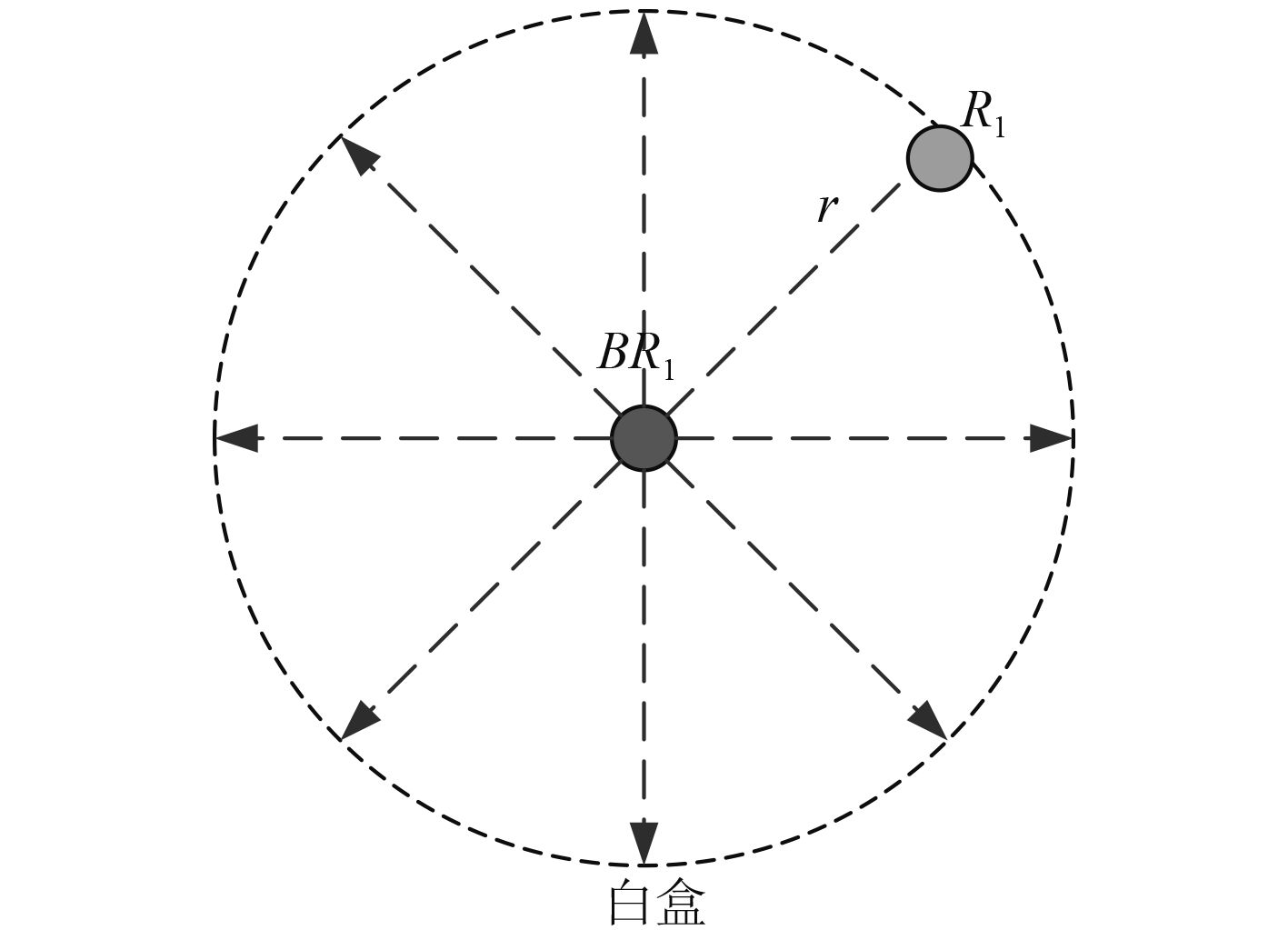图 1 白盒模型 Fig. 1 No-box model

2.2.2 黑盒模型

 ${P_{R2}} = \frac{{{P_0}}}{{2π {r^2}}}\text{，}$
 ${P_{R1}} = 0\text{。}$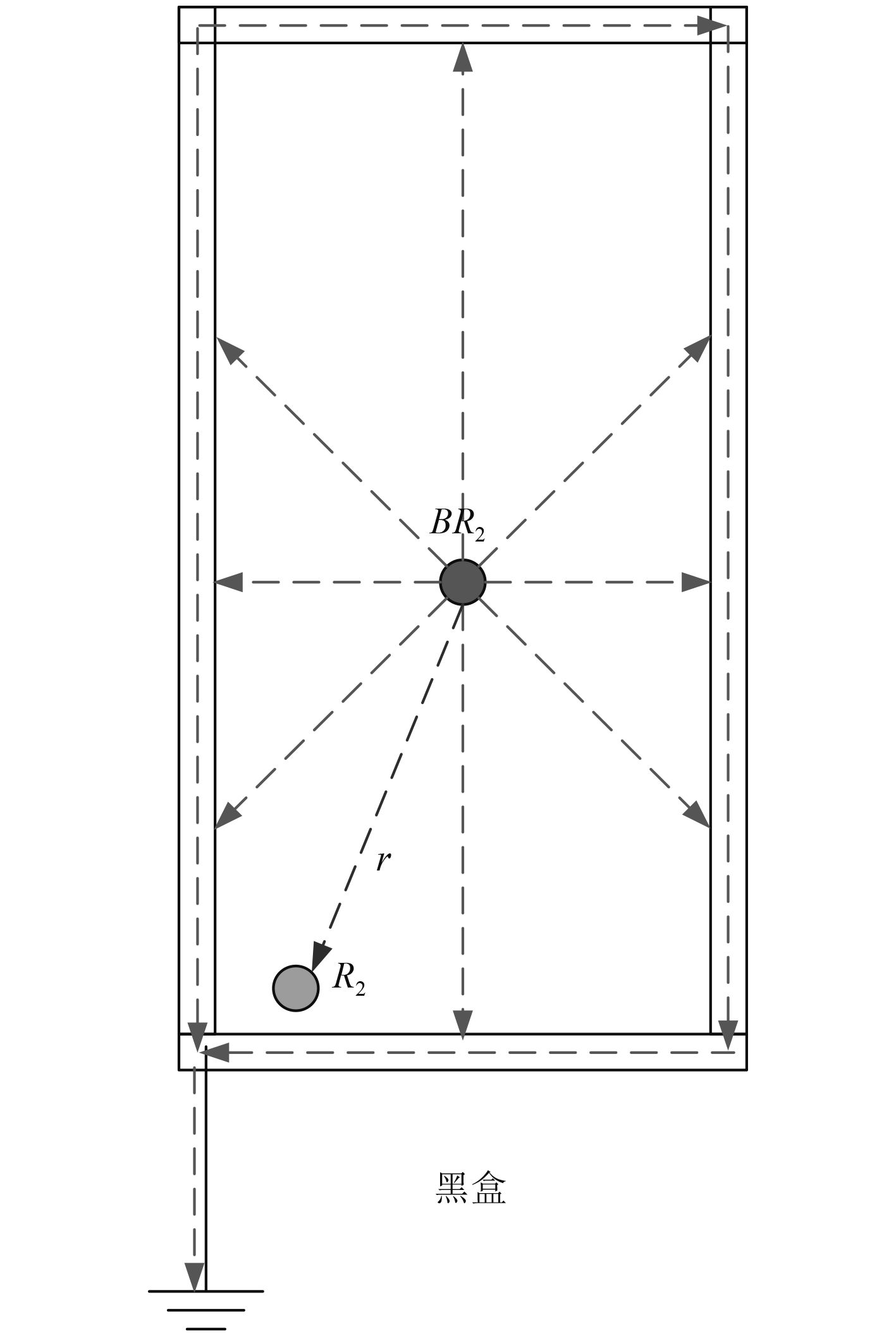图 2 黑盒模型 Fig. 2 Closed-box model
2.2.3 窗口模型

 ${P_{R3}} = \frac{{{P_0}}}{{2π {r^2}}}\text{，}$
 ${P_{R4}} = 0\text{，}$
 ${P_{R5}} = \frac{{{P_0}}}{{2π {r_5}^2}}\text{。}$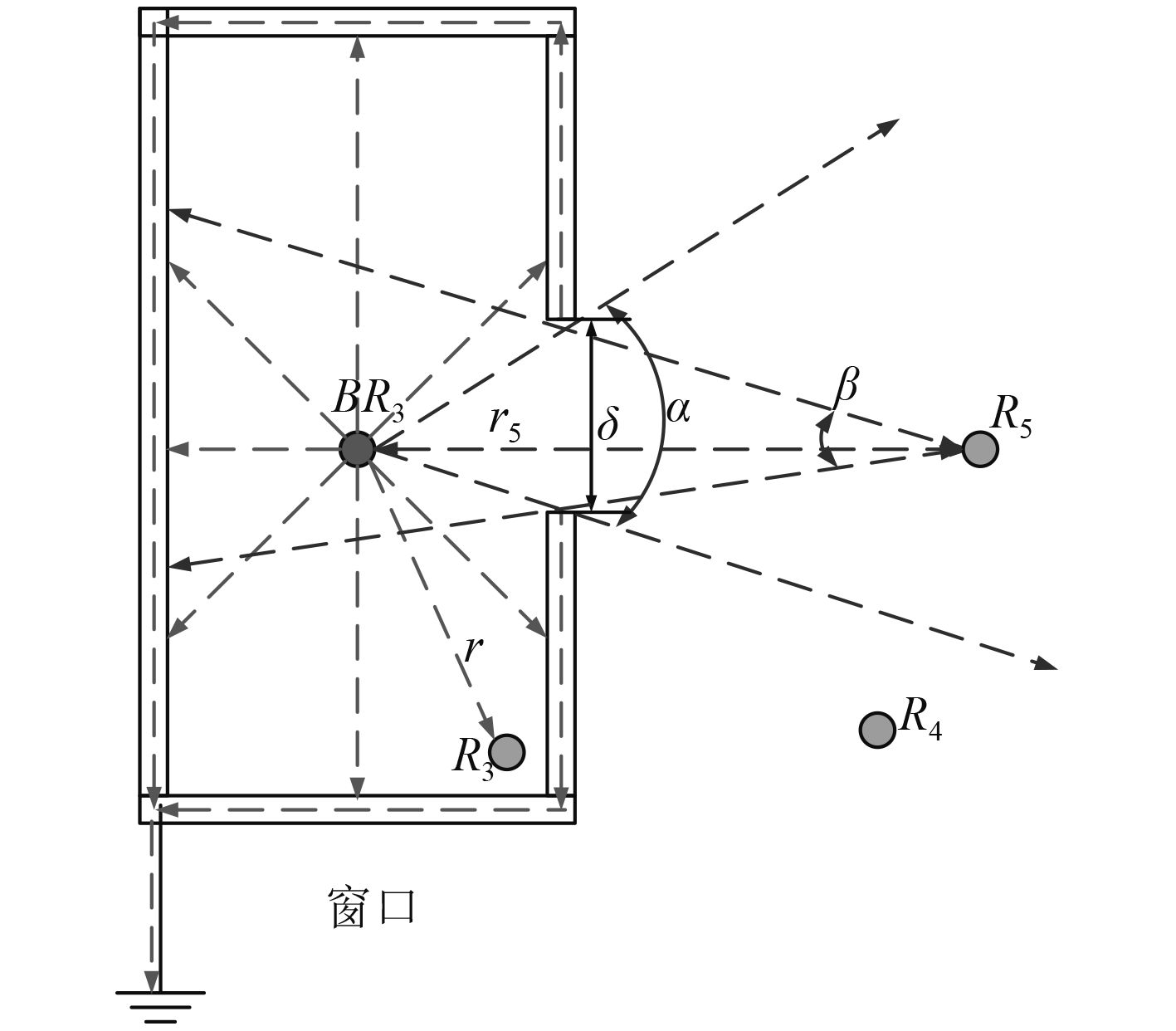图 3 窗口模型 Fig. 3 Open-box model

2.2.4 反射模型

 ${P_{R6}} = \frac{{{P_0}}}{{2π {c^2}}}\text{。}$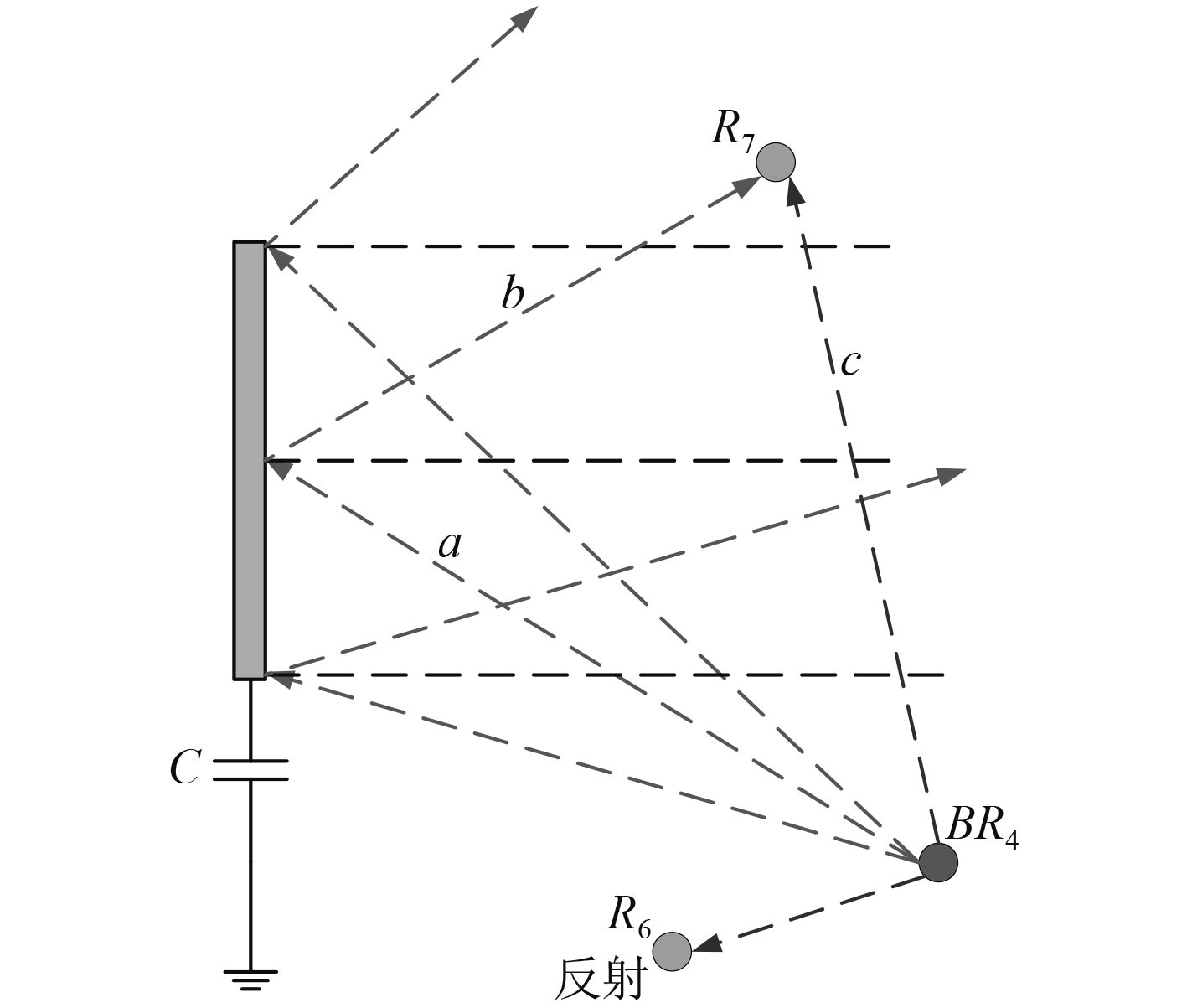图 4 反射模型 Fig. 4 Reflection-interference model

 \begin{aligned}{\vec E_{R7}} = & \overrightarrow {{E_c}} + {\vec E_{\left( {a + b} \right)}} = {A_C}\cos \left[ {\omega t + \left( {{\psi _c} - \frac{{2π c}}{\lambda }} \right)} \right] + \\ &{A_{\left( {a + b} \right)}}\cos \left[ {\omega t + \left( {{\psi _{\left( {a + b} \right)}} - \frac{{2π \left( {a + b} \right)}}{\lambda }} \right) - π } \right]\text{。}\end{aligned}

2.2.5 衍射模型

 ${P_{R8}} = \frac{{{P_0}}}{{2π {r_1}^2}} \times \frac{1}{{2π r_2^2}} = \frac{{{P_0}}}{{4{π ^2}r_1^2r_2^2}}\text{。}$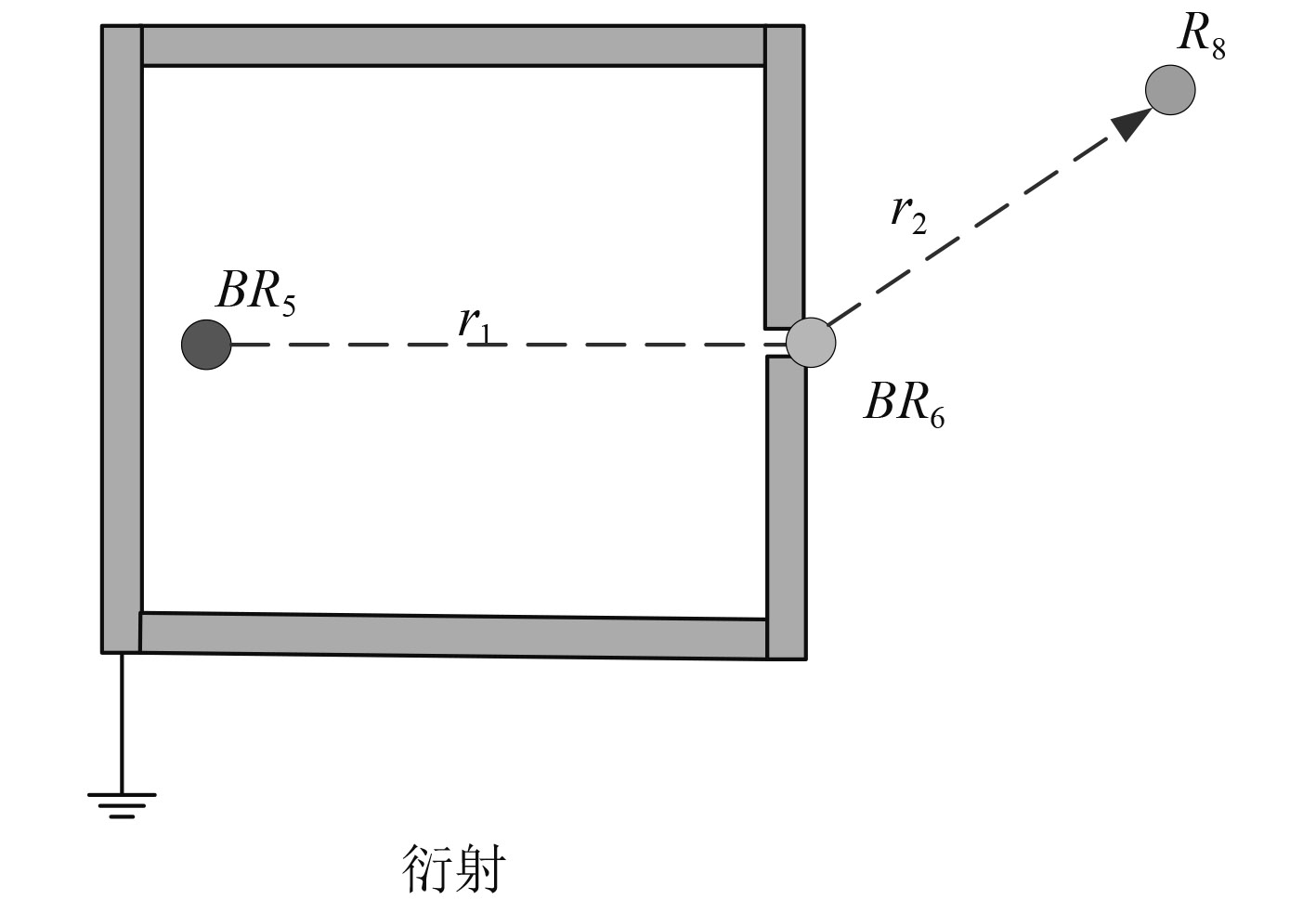图 5 衍射模型 Fig. 5 Diffraction-interference model

2.2.6 地面模型

 ${P_{R9}} = \frac{{{P_0}}}{{2π {r^2}}}\text{。}$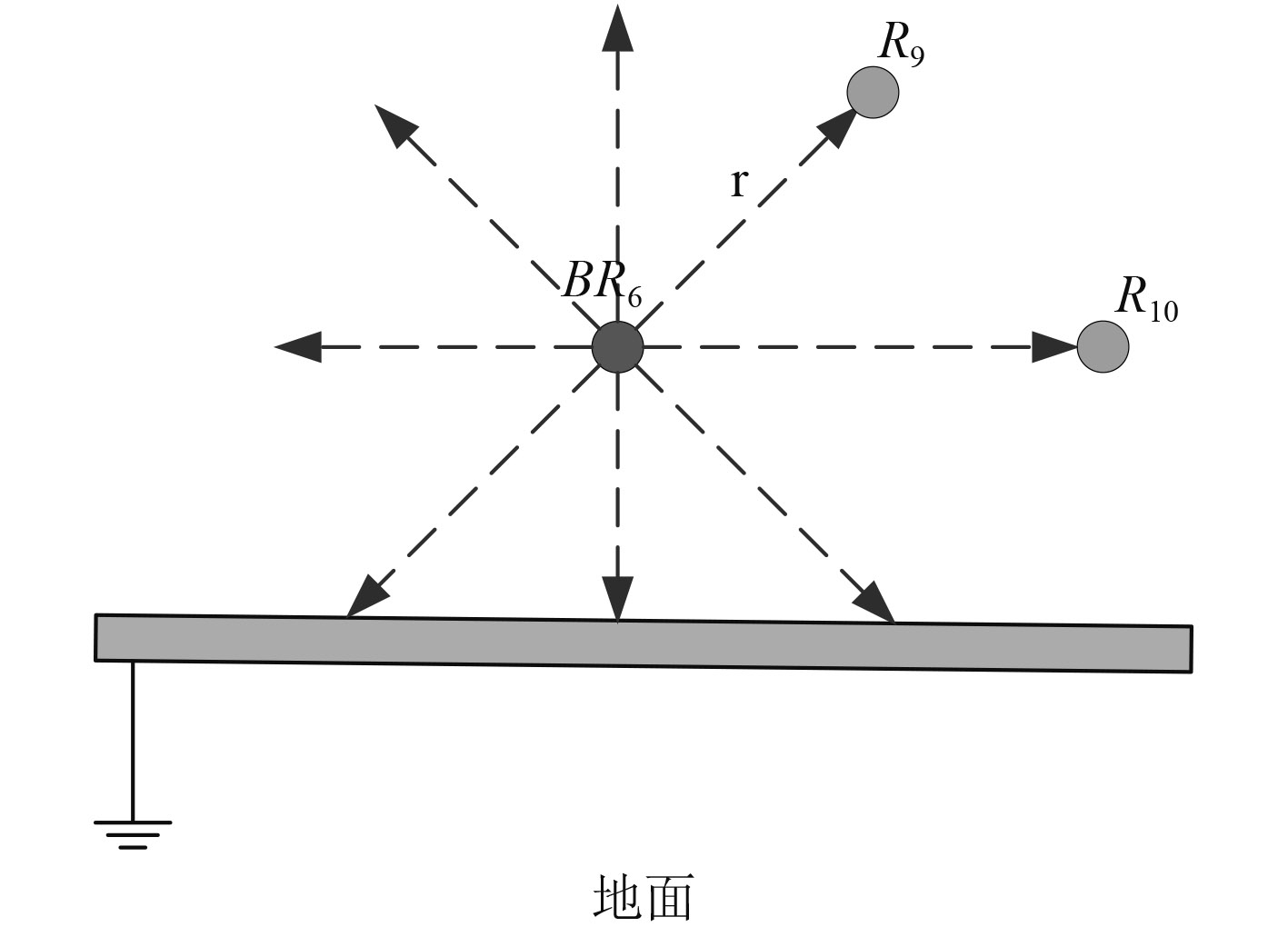图 6 地面模型 Fig. 6 Ground-interference model
2.3 动态组网模型建立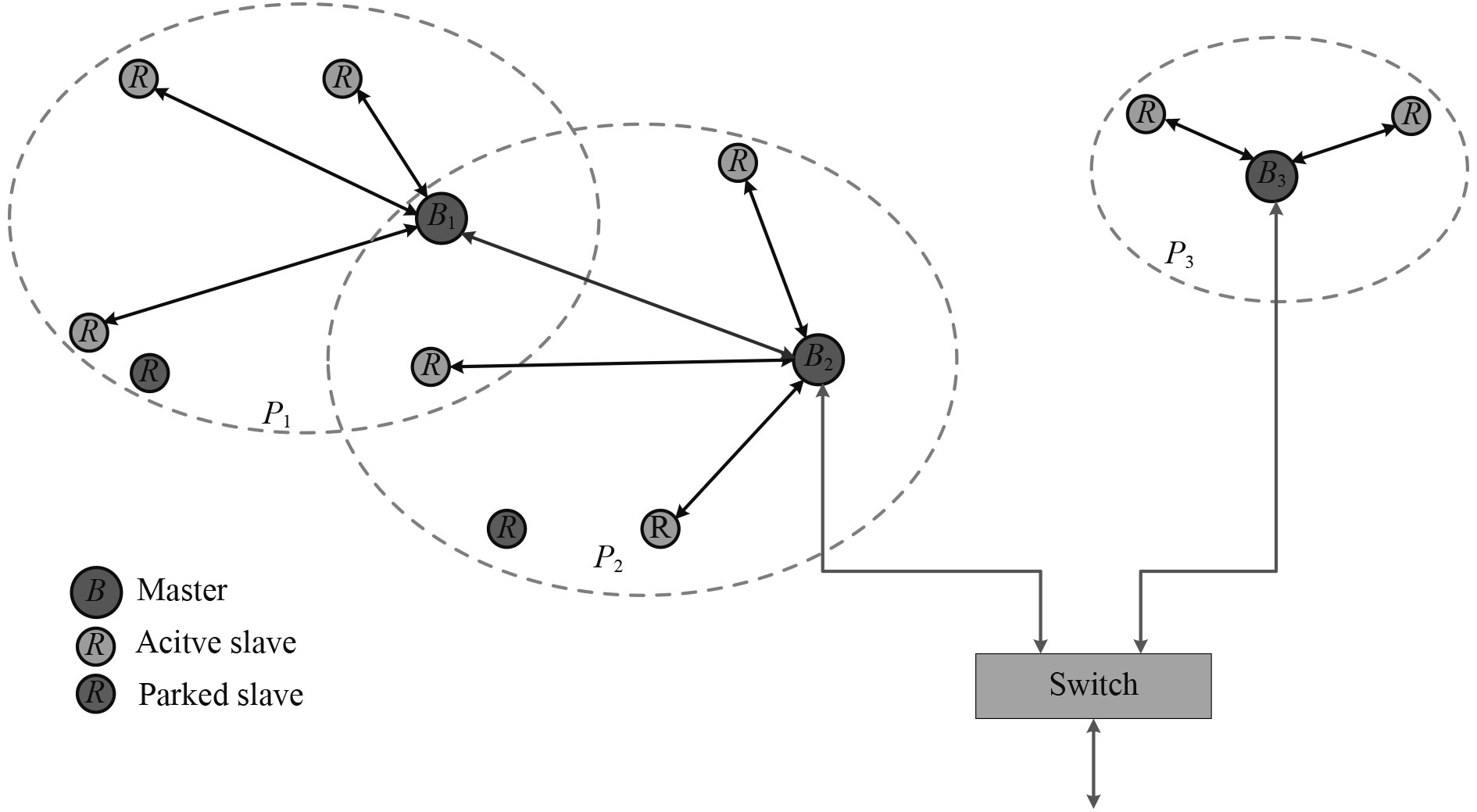图 7 混合网络 Fig. 7 Hybrid network
2.3.1 微微网

2.3.2 散射网

2.3.3 混合网

${v_{mn0}}$ Rn在距离Bm为1m时测量的RSSI基准值，ω 为环境衰减因子，则

 ${\mu _{\left( {m,n} \right)}} = \frac{{{v_{mn}}}}{{256}}\text{，}$
 ${\gamma _{\left( {m,n} \right)}} = {10^{\frac{{abs\left( {{v_{mn}}} \right) - {v_{mn0}}}}{{10 \times \omega }}}}\text{，}$
 $U = \left\{ {\begin{array}{*{20}{c}}{{\mu _{\left( {1,1} \right)}}}& \ldots &{{\mu _{\left( {1,N} \right)}}}\\ \vdots & \ddots & \vdots \\{{\mu _{\left( {M,1} \right)}}}& \ldots &{{\mu _{\left( {M,N} \right)}}}\end{array}} \right\}\text{。}$

 ${{ F}_\lambda } = \left[ {\begin{array}{*{20}{c}}{1,1,0,0}& \cdots &0\\ \vdots & \ddots & \vdots \\{1,1,1,1}& \cdots &0\end{array}} \right]\text{。}$

3 船舶WSN组网优化对策

1）WSN固定节点安装位置不影响船舶功能的使用，例如不能影响人员活动；

2）WSN固定节点安装充分考虑无线电传输效能和覆盖面；

3）WSN固定节点本身防护，例如最高支持IP66防护、防雷、防盗、防拆解；

4）WSN移动节点本身防护，例如最高支持IP67防护、支持无线充电

3.1 首尾甲板

3.2 平台甲板

3.3 船舷走廊

3.4 内部走廊

3.5 办公生活舱室

3.6 机舱

3.7 高频设备间

3.8 窗户和窄缝

4 结 语

  中国船级社. 智能船舶规范2015[S]. http://www.moc.gov. cn/zizhan/zhishuJG/chuanjishe/guifanzhinan/201512/P020151202371212558498.pdf China Classification Societf. Rules for Intelligent Ships (2015) [S].  李铭志, 柳存根, 蒋如宏, 赵永生, 马宁, 等. 船舶电气智能设计数字化信息模型研究[J]. 中国造船, 2011 (1): 186–192. LI Ming-zhi, LIU Cun-gen, JIANG Ru-hong, ZHAO Yongsheng, MA Ning, et al. Study on the digitize information model in intelligent marine electric design system[J]. Shipbuilding of China, 2011 (1): 186–192.  江正, 刘辉, 向先波. 基于C/S架构的船舶电站网络化无线监控系统设计[J]. 中国造船, 2015 (4): 179–187. JIANG Zheng, LIU Hui, XIANG Xianbo. Wireless network monitoring system for ship power station based on C/S architecture[J]. Shipbuilding of China, 2015 (4): 179–187.  上海海事大学. 基于WSN构建的船舶驾驶台火灾监控系统[P]. CN 102083169 A.2011.06.01.  上海海事大学. 一种基于WSN及INMARSAT-F 的船舶安全远程实时监控系统[P]. CN102083169A.2010.04.15.  张晓平. WSN移动目标的LSSVR回归建模定位理论与算法[D]. 广州: 华南理工大学 2010. ZHANG Xiao-ping. Study on theory and algorithm of mobile target localization in WSN based on LSSVR regression modeling[D]. Guang zhou: South China University of Technology, 2010.  温家旺. 复杂条件下的无线传感器网络定位技术研究[D]. 南京: 南京航空航天大学, 2014. WEN Jia-wang. Research on the localization technology in wireless sensor network under complex conditions[D]. Nan jing: Nanjing University of Aeronautics and Astronautics. 2014.  张勇, 史雅楠, 黄杰, 李飞腾, 等. 基于独立成分分析与核典型相关分析的WLAN室内定位方法[J]. 计算机应用研究, 2016, 12 : 3817–3821. ZHANG Yong, SHI Yanan, HUANG Jie, LI Feiteng. WLAN indoor positioning algorithms based on independent component analysis and kernel canonical correlation analysis[J]. Application Research of Computers, 2016, 12 : 3817–3821. DOI: 10.3969/j.issn.1001-3695.2016.12.066  覃立中. 多径反射对WSN节点测距精确度影响的分析与对策[D]. 哈尔滨: 哈尔滨工业大学, 2014. QIN Li-zhong. Analysis and countermeasures about multipath reflections on the impact of WSN nodes ranging accuracy[D]. Harbin: Harbin Institute of Technology Master’s Thesis, 2014.  宗立成, 叶聪, 余隋怀, 陈登凯, 等. 载人潜水器舱室设备智能布局设计方法研究[J]. 中国造船, 2013 (3): 147–154. ZONG Licheng, YE Cong, YU Suihuai, CHEN Dengkai, et al. Research and Application of Intelligent Layout Method in DSV Cabin Equipment[J]. Shipbuilding of China, 2013 (3): 147–154.  李振荣. 基于蓝牙的无线通信芯片关键技术研究[D]. 西安: 西安电子科技大学 2010. LI Zhen-rong. Key technology research of wireless communication chip based on bluetooth[D]. Xian: Xidian University. 2010.  汪金菊. 贝叶斯模型及其在混沌序列分析中的应用研究[D]. 合肥: 合肥工业大学, 2008. WANG Jin-ju. Resear chon bayesian model swith APP lieationto chaotic time serie analysis[D]. Hefei: University of Technology, 2008.  丁贤根. 带磁隔离和电隔离及天线的抗EMI线路板[P]. 201611003437.6.2016.11.16.  丁贤根. 民营IT企业期权激励对员工满意度影响的研究[D]. 上海: 复旦大学 2013.  卢中昊. 系统级电磁兼容现场测量关键技术研究[D]. 长沙: 国防科学技术大学 2013. LU Zhong-hao. Studies on key techniques of in-situ test for system level electromagnetic compatibility[D]. Changsha: Graduate School of National University of Defense Technology. 2013.  张志柏. 应用于复杂电磁环境下的船舶自动通信系统研究[J]. 应用于复杂电磁环境下的船舶自动通信系统研究[J]. 舰船科学技术, 2016 (3A): 139–141. ZHANG Zhi-bai. Research on the automatic communication system of ships in the complex electromagnetic environment[J]. Ship Science and Technology, 2016 (3A): 139–141.  李光所 舰船电磁环境预测分析方法[J]. 舰船电磁环境预测分析方法[J]. 船舶电气, 2010 (5): 41–43. Li Guangsuo. Predictive analysison shipboard electromagnetic environment[J]. Ship & Boad, 2010 (5): 41–43.